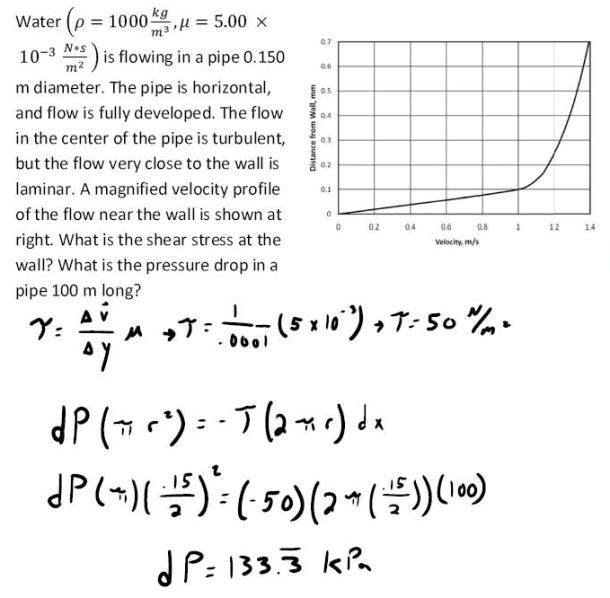fluid mechanics math problems engineering equations fluid mechanics basic mechanics school homework engineering math fluids formulas fluid mechanics problems fluid mechanics problem solutions to fluids problems full solution fluid mechanics engineering fluids problem solution fluid mechanics math problems engineering equations fluid mechanics basic mechanics school homework engineering math fluid mechanics formulas fluid problems fluid mechanics problem solutions to fluid mechanics problems full solution fluid mechanics engineering fluid mechanics problem solution
fluid mechanics math problems engineering equations fluid mechanics basic mechanics school homework engineering math fluids formulas fluid mechanics problems fluid mechanics problem solutions to fluids problems full solution fluid mechanics engineering fluids problem solution fluid mechanics math problems engineering equations fluid mechanics basic mechanics school homework engineering math fluid mechanics formulas fluid problems fluid mechanics problem solutions to fluid mechanics problems full solution fluid mechanics engineering fluid mechanics problem solution
Highalphabet Home Page fluid mechanics solutions fluids math solved Fluid Mechanics Page 1
Water is flowing in a pipe .15 m diameter. The pipe is horizontal, and flow is fully developed. The flow in the center of the pipe is turbulent, but the flow very close to the wall is laminar. A magnified velocity profile of the flow near the wall is whon at right. What is the shear stess at the wall? What is the pressure drop in a pipe 100 m long?Water is flowing in a pipe .15 m diameter. The pipe is horizontal, and flow is fully developed. The flow in the center of the pipe is turbulent, but the flow very close to the wall is laminar. A magnified velocity profile of the flow near the wall is whon at right. What is the shear stess at the wall? What is the pressure drop in a pipe 100 m long?